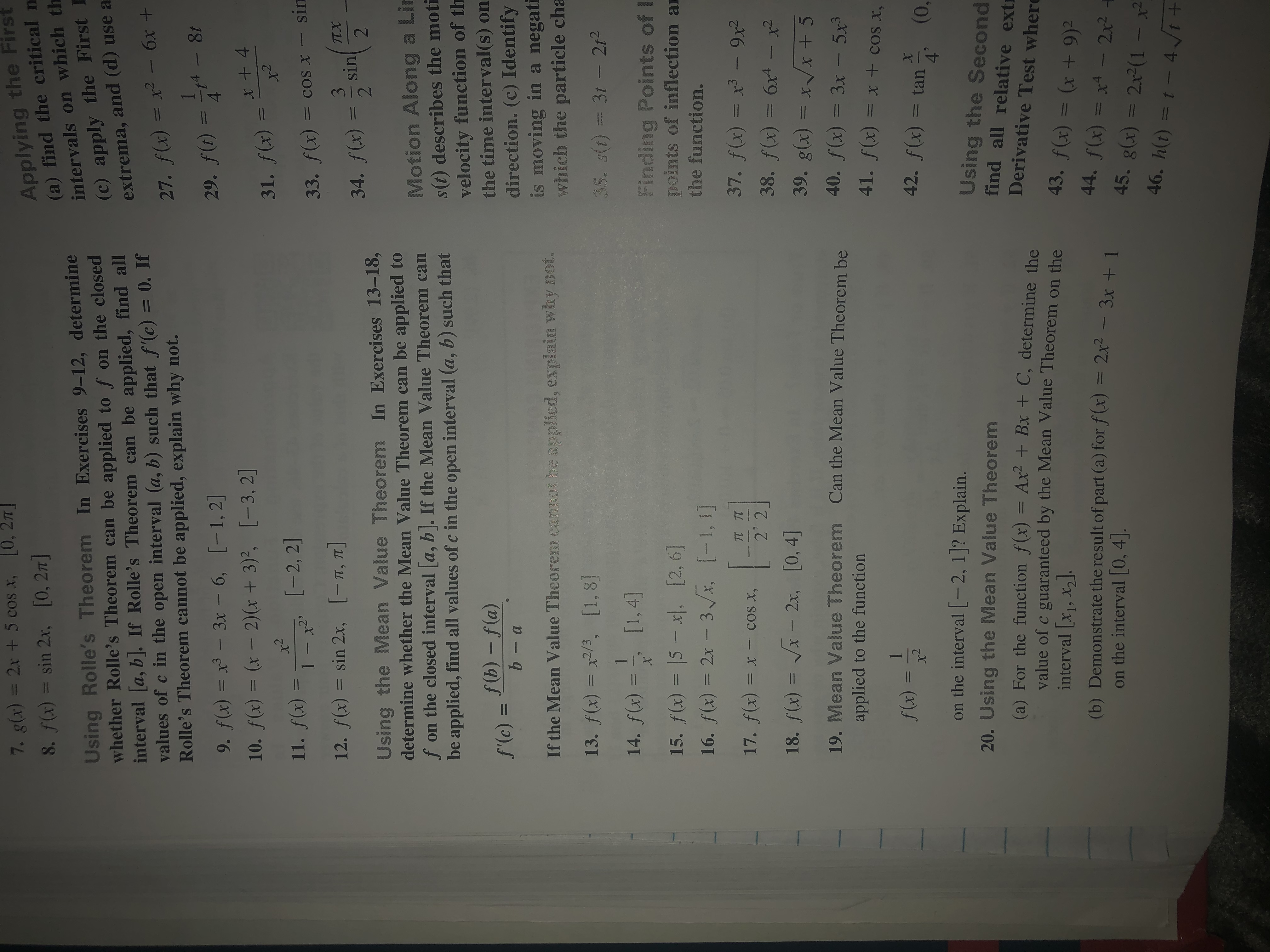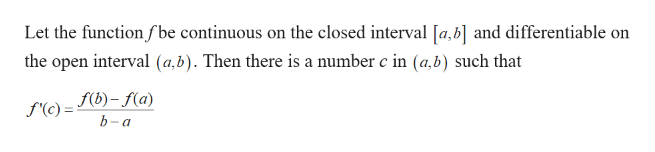# Applying the First(a) find the critical nintervals on which th(c) apply the First7. g(x) = 2x + 5 cos x, 0, 278. f(x) = sin 2x, [0, 27]Using Rolle's Theorem In Exercises 9-12, determinewhether Rolle's Theorem can be applied to f on the closedinterval a, b. If Rolle's Theorem can be applied, find allvalues of c in the open interval (a, b) such that f'(c) = 0. IfRolle's Theorem cannot be applied, explain why not.and (d) use aextrema,бх +27. f(x) = x2 -6x8t29. f()49. f(x) = x3 - 3x - 6, [-1, 2]x+410. f(x) = (x- 2) (x + 3)2, [-3, 2]31. f(x) =[-2, 2]11. f(x)sin1 - x2'33. f(x) = coSsin12. f(x) = sin 2x, [-T, T]3TX34. f(x)22Using the Mean Value Theorem In Exercises 13-18,determine whether the Mean Value Theorem can be applied tof on the closed interval a, b. If the Mean Value Theorem canbe applied, find all values of c in theMotion Along a Lins(t) describes the motivelocity function of ththe time interval (s) ondirection. (c) Identifyis moving in a negatiwhich the particle chaopen interval (a, b) such thatf (b) - f(a)b- af'(c) =If the Mean Value Theorem canpot be applied, explain why not.13. f(x) x2/3, [1, 835. sid = 3t -2121[1, 4]14. f(x)Finding Points of Ipoints of inflection a15. f(x) = 15 - x| [2,6]3x. [-1, 1]the function.16. f(x) = 2x37. f(x) = x 9x238. f(x) = 6x4 -39. g(x) = x x +540. f(x) 3x -5x341. f(x)17. f(x) = x- cos x,2' 2x218. f(x) = x 2x, [0,4]19. Mean Value TheoremЗх —Can the Mean Value Theorem beapplied to the function=XtcoSx,1f(x) =42. f(x) ==tan(0,4on the interval [-2, 1]? Explain.Using the Secondfind all relative extr20. Using the Mean Value Theorem(a) For the function f(x) Ax Bx + C, determine thevalue of c guaranteed by the Mean Value Theorem on theinterval [xj, x2](b) Demonstrate the result of part (a) for f(x) = 2x2 - 3x +1on the interval 0, 4]Derivative Test where43. f(x) = (x +9)244. f(x) = x4 - 2x245. g(x) = 2x2(1- x246. h(t) = t - 4 t+11

Question
45 views

19help_outlineImage TranscriptioncloseApplying the First (a) find the critical n intervals on which th (c) apply the First 7. g(x) = 2x + 5 cos x, 0, 27 8. f(x) = sin 2x, [0, 27] Using Rolle's Theorem In Exercises 9-12, determine whether Rolle's Theorem can be applied to f on the closed interval a, b. If Rolle's Theorem can be applied, find all values of c in the open interval (a, b) such that f'(c) = 0. If Rolle's Theorem cannot be applied, explain why not. and (d) use a extrema, бх + 27. f(x) = x2 -6x 8t 29. f() 4 9. f(x) = x3 - 3x - 6, [-1, 2] x+4 10. f(x) = (x- 2) (x + 3)2, [-3, 2] 31. f(x) = [-2, 2] 11. f(x) sin 1 - x2' 33. f(x) = coS sin 12. f(x) = sin 2x, [-T, T] 3 TX 34. f(x) 2 2 Using the Mean Value Theorem In Exercises 13-18, determine whether the Mean Value Theorem can be applied to f on the closed interval a, b. If the Mean Value Theorem can be applied, find all values of c in the Motion Along a Lin s(t) describes the moti velocity function of th the time interval (s) on direction. (c) Identify is moving in a negati which the particle cha open interval (a, b) such that f (b) - f(a) b- a f'(c) = If the Mean Value Theorem canpot be applied, explain why not. 13. f(x) x2/3, [1, 8 35. sid = 3t -212 1 [1, 4] 14. f(x) Finding Points of I points of inflection a 15. f(x) = 15 - x| [2,6] 3x. [-1, 1] the function. 16. f(x) = 2x 37. f(x) = x 9x2 38. f(x) = 6x4 - 39. g(x) = x x +5 40. f(x) 3x -5x3 41. f(x) 17. f(x) = x- cos x, 2' 2 x2 18. f(x) = x 2x, [0,4] 19. Mean Value Theorem Зх — Can the Mean Value Theorem be applied to the function =XtcoSx, 1 f(x) = 42. f(x) = =tan (0, 4 on the interval [-2, 1]? Explain. Using the Second find all relative extr 20. Using the Mean Value Theorem (a) For the function f(x) Ax Bx + C, determine the value of c guaranteed by the Mean Value Theorem on the interval [xj, x2] (b) Demonstrate the result of part (a) for f(x) = 2x2 - 3x +1 on the interval 0, 4] Derivative Test where 43. f(x) = (x +9)2 44. f(x) = x4 - 2x2 45. g(x) = 2x2(1 - x2 46. h(t) = t - 4 t+ 11 fullscreen
check_circle

Step 1

Mean Value Th...help_outlineImage TranscriptioncloseLet the function fbe continuous on the closed interval [a,b] and differentiable on the open interval (a,b). Then there is a number c in (a,b) such that fb)-f(a) f'(c) = b-а fullscreen

### Want to see the full answer?

See Solution

#### Want to see this answer and more?

Solutions are written by subject experts who are available 24/7. Questions are typically answered within 1 hour.*

See Solution
*Response times may vary by subject and question.
Tagged in

### Continuity